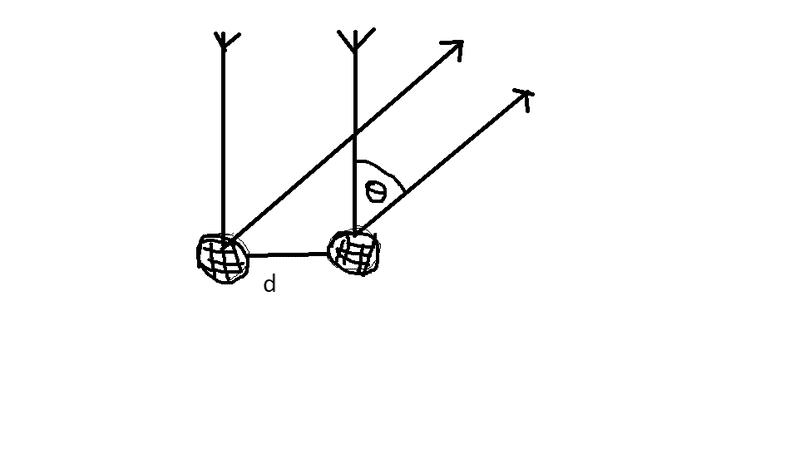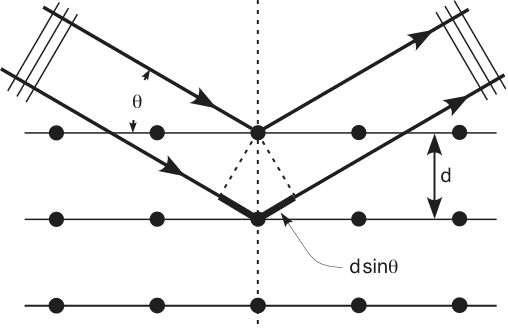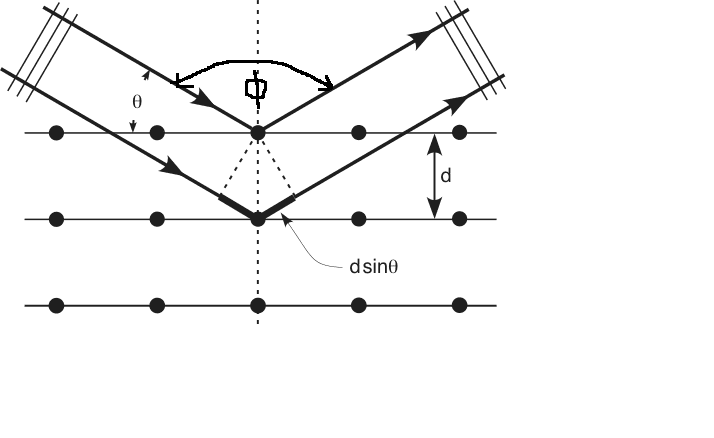# De Broglie wavelength neutrons

Question-A certain crystal has a set of planes spaced 0.376 nm apart. A beam of neutrons strikes the crystal at normal incidence and the first maximum of the diffraction pattern occurs at a scattering angle (i.e angle between incoming neutrons and reflected beam) of 41.4 degrees. What is the de Broglie wavelength of the neutrons in units nm and to 3 decimal places.

Usefull equations -
2d sin theata = nλ
where d = plane spaceing, n is the order in this case =1

My Attempt-
useing above eqn,
2*0.0376E-9 (m)*sin(41.4)=4.973E-11 m

I have been told that this solution is wrong as the question states "at normal incidence" which apparantly complicates things and makes a need to change the angle theata. Any suggestions? thanks in advance.

Right, real simple. Try drawing a picture and then revisit what they mean by "angle between incoming neutrons and reflected beam" and make sure you drew it correctly.

You're almost there.

yeah its the drawing which is what is stuffing me up. when i draw a picture i have the neutrons comeing in at 90 degrees to the plane ie normal and the reflecting out at 41.4 degrees. but i dont think this is right?Perform an internet search for "Bragg Diffraction."Well done. Now think on that for a bit.

Looking at the question again it states that the neutrons are hitting the plane normal ie at theata = 90 degrees which is the dotted line on that diagram right. Also we know the first maximum of the diffraction pattern occurs at a scattering angle (i.e angle between incoming neutrons and reflected beam) of 41.4 degrees.
So does this mean that as theata is measured with respect to the plane, theata is 90-41.4?

Excellent! That's the second step. We're missing the first one. Nearly there. Think about where the "angle between incoming neutrons and reflected beam" is.well the angle (phi) is the angle between incomming neutrons and reflected beem, and in our case phi= 41.4 degrees. So what am i missing? thank you very much for your patience.

No troubles. You've done quite well. Great picture. I believe you're a good student for being so patient yourself.

Answer: We need to halve it.

Then do what you were saying.

I see, so we have 90-1/2phi
=90-20.7
=69.3 degress
and from this we can plug theata into the 2dsintheata=nlambda
correct?

Time to celebrate.

thank you very much.